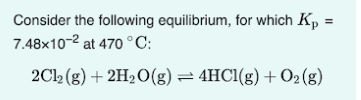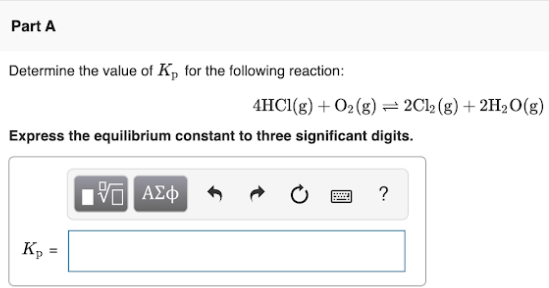# Consider the following equilibrium, for which Kp = 7.48x10^-2 at 470 °C: 2Cl2(g) + 2H2O(g) ⇌ 4 HCl(g) + O2(g) Determine the value of Kp for the following reaction: 4HCl(g) + O2(g) ⇌ 2Cl2(g) + 2H2O(g) Express the equilibrium constant to three significant digits.